# Quiz 2: The Market System and the Circular Flow

Suppose that there are three techniques for producing \$15 worth of bar soap. The cost/price of each bar soap is \$3 bar and each of the technique is producing 5 bar soaps. (a)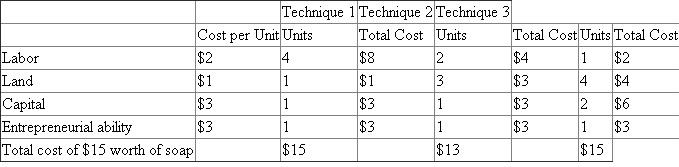The above table shows the cost of making \$15 worth of soap using three different techniques that require different amounts of inputs of labor, land, capital, and entrepreneurial ability. It is given that each bar of soap is worth \$3.00, which means 5 bars of soap is worth \$15. Note that technique 2 is the best way to make 5 bars of soap because it can make \$15 worth of soap for \$13. If the price of a single bar of soap falls to \$2.75, the firm can earn revenue of \$13.75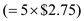if 5 bars are sold. This means technique 1 and technique 3 will actually cause to lose money (since the price of bar soaps falls which yield \$13.75 of revenue but these two costs \$15 to make). Technique 2 is the only way you can make soap without losing money because it is making soap bars at cost \$13 which is less than \$13.75. If the price of soap was instead \$4 or \$5, it will recommendable to use technique 2 because it is the most cost-effective way to make soap with the current input prices. Although one can now make a profit now using technique 1 or 3, but one can have higher profit using technique 2 because it makes soap at a lower cost. (b) If the price of soap fell to \$2, it is not worth it to make any soap. Even using technique 2, which is the technique with the lowest cost, each bar of soap cost you \$13/5=\$2.60. If each bar of soap cost you \$2.60 to make and you can only sell it for \$2.00, you will not even want to make a single bar of soap, because doing so would make you lose money. (c) Suppose now each bar of soap is \$3.00 and the cost of each resource is \$1. Our table now looks like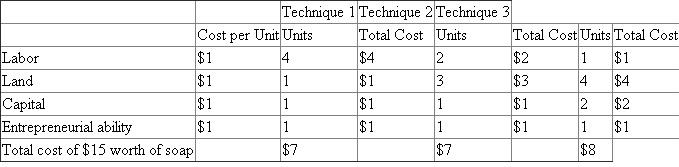The table is showing the new total cost of all three techniques. The total cost is calculated by multiplying per unit cost with the number of units and then summing up to get the final total cost. The least profitable technique is now technique 3 , since it cost \$8 when both technique 1 and technique 2 cost \$7. (d) Suppose now resource prices are back to the original price, but there is a technique 4 that can produce 3 bars of soap using 1 unit of each resource. With each unit of labor, land, capital, and entrepreneurial ability costing \$2, \$1, \$3, and \$3 respectively, the cost to make three bars of soap using technique 4 is \$9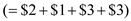. That means each bar of soap cost \$3 to make. This means that technique 4 is as cost effective as technique 1 anD3, because techniqueS1 anD3 both make 5 bars of soap for \$15 (which means it cost \$3 to make each bar of soap). Technique 2, however, can make 5 bars of soap for \$13, which is \$2.60 per bar. Because technique 2 is more cost effective, companies will not prefer the new technique.
Two sector circular flow model represents the real flow of goods and services and monetary flow of income and expenditure taking place between households and businesses. Households are expected to provide factor services to businesses in resource market in exchange for factor income. The same income is then used to purchase the goods and services sold by businesses in product market. Figure 1 illustrates the economy with two sectors only: households and businesses. The representative circular model shows the inner flow of goods and services and the outer flow of money between these two sectors.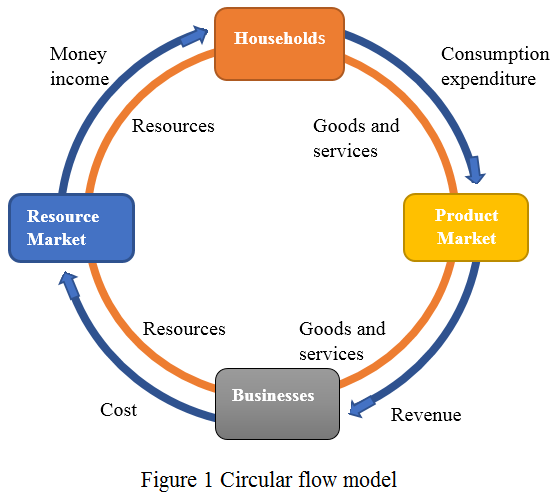The model described in Figure 1 exhibits two flows in the resource market. The first one has households providing factor services to businesses which is then used to manufacture goods and services. The second one has businesses paying factor income to households for rendering their factor services. In this manner, household are selling their factor services in the resource market while the businesses are purchasing them. Hence,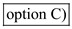is correct.